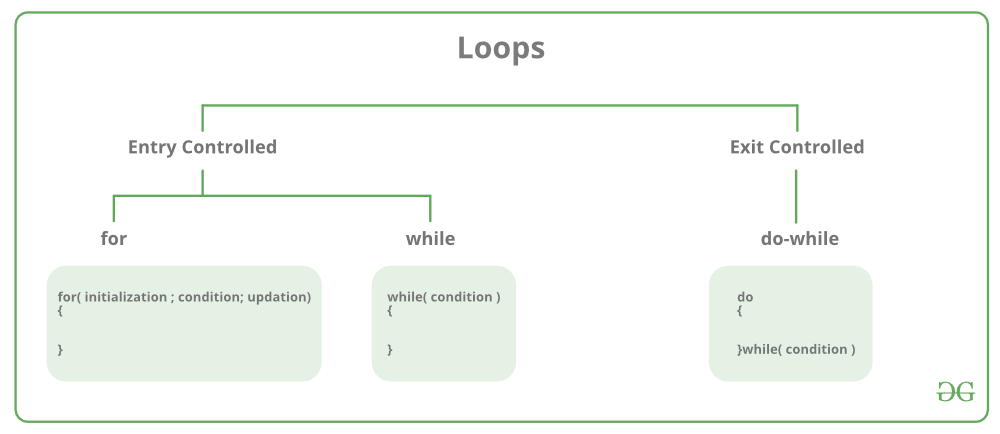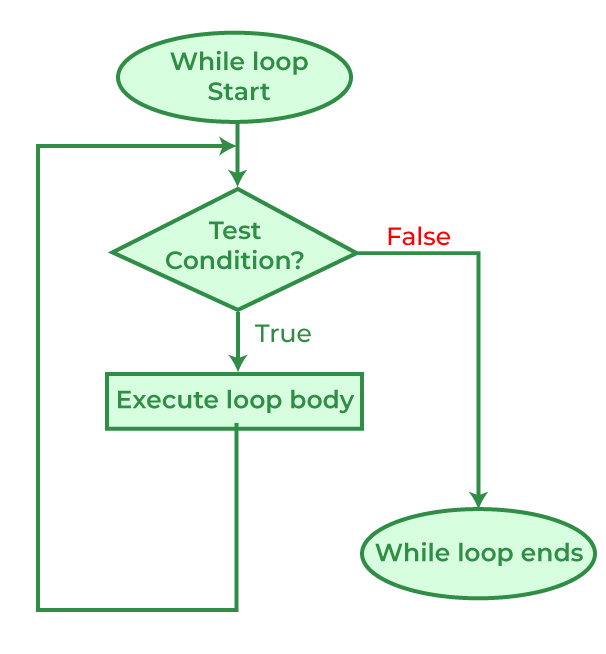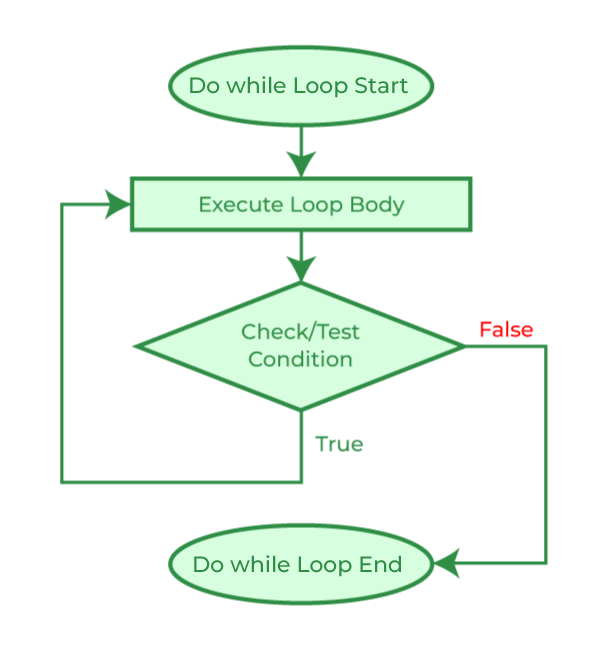Open in App
Not now

## Related Articles

• Write an Interview Experience
• CBSE Class 11 Syllabus
• CBSE Class 11 Maths Notes
• CBSE Class 11 Physics Notes
• CBSE Class 11 Biology Notes
• CBSE Class 11 | Computer Science – C++ Syllabus

# C++ Loops

• Difficulty Level : Easy
• Last Updated : 18 Mar, 2023

In Programming, sometimes there is a need to perform some operation more than once or (say) n number of times. Loops come into use when we need to repeatedly execute a block of statements.

For example: Suppose we want to print “Hello World” 10 times. This can be done in two ways as shown below:

### Manual Method (Iterative Method)

Manually we have to write cout for the C++ statement 10 times. Let’s say you have to write it 20 times (it would surely take more time to write 20 statements) now imagine you have to write it 100 times, it would be really hectic to re-write the same statement again and again. So, here loops have their role.

## C++

 `// C++ program to Demonstrate the need of loops``#include ``using` `namespace` `std;` `int` `main()``{``    ``cout << ``"Hello World\n"``;``    ``cout << ``"Hello World\n"``;``    ``cout << ``"Hello World\n"``;``    ``cout << ``"Hello World\n"``;``    ``cout << ``"Hello World\n"``;``    ``return` `0;``}`

Output

```Hello World
Hello World
Hello World
Hello World
Hello World```

Time complexity: O(1)

Space complexity: O(1)

### Using Loops

In Loop, the statement needs to be written only once and the loop will be executed 10 times as shown below.  In computer programming, a loop is a sequence of instructions that is repeated until a certain condition is reached.

## There are mainly two types of loops:

1. Entry Controlled loops: In this type of loop, the test condition is tested before entering the loop body. For Loop and While Loop is entry-controlled loops.
2. Exit Controlled Loops: In this type of loop the test condition is tested or evaluated at the end of the loop body. Therefore, the loop body will execute at least once, irrespective of whether the test condition is true or false. the do-while loop is exit controlled loop.## For Loop-

A For loop is a repetition control structure that allows us to write a loop that is executed a specific number of times. The loop enables us to perform n number of steps together in one line.

Syntax:

```for (initialization expr; test expr; update expr)
{
// body of the loop
// statements we want to execute
}```

### Explanation of the Syntax:

• Initialization statement: This statement gets executed only once, at the beginning of the for loop. You can enter a declaration of multiple variables of one type, such as int x=0, a=1, b=2. These variables are only valid in the scope of the loop. Variable defined before the loop with the same name are hidden during execution of the loop.
• Condition: This statement gets evaluated ahead of each execution of the loop body, and abort the execution if the given condition get false.
• Iteration execution: This statement gets executed after the loop body, ahead of the next condition evaluated, unless the for loop is aborted in the body (by break, goto, return or an exception being thrown.)

### NOTES:

• The initialization and increment statements can perform operations unrelated to the condition statement, or nothing at all – if you wish to do. But the good practice is to only perform operations directly relevant to the loop.
• A variable declared in the initialization statement is visible only inside the scope of the for loop and will be released out of the loop.
• Don’t forget that the variable which was declared in the initialization statement can be  modified during the loop, as well as the variable checked in the condition.

Example1:

```for(int i = 0; i < n; i++)
{
// BODY
}```

Example2:

```for(auto element:arr)
{
//BODY
}```

### Flow Diagram of for loop:Example1:

## C++

 `// C++ program to Demonstrate for loop``#include ``using` `namespace` `std;` `int` `main()``{``    ``for` `(``int` `i = 1; i <= 5; i++) {``        ``cout << ``"Hello World\n"``;``    ``}` `    ``return` `0;``}`

Output

```Hello World
Hello World
Hello World
Hello World
Hello World```

Time complexity: O(1)

Space complexity: O(1)

## C++

 `#include ``using` `namespace` `std;` `int` `main() {` `int` `arr[] {40, 50, 60, 70, 80, 90, 100};`` ``for` `(``auto` `element: arr){``   ``cout << element << ``" "``;`` ``}``return` `0;` `}`

Output

`40 50 60 70 80 90 100 `

Time complexity: O(n) n is the size of array.

Space complexity: O(n) n is the size of array.

## C++

 `#include ``using` `namespace` `std;` `int` `main()``{``    ``for` `(``int` `i = 0, j = 10, k = 20; (i + j + k) < 100;``         ``j++, k--, i += k) {``        ``cout << i << ``" "` `<< j << ``" "` `<< k << ``"\n"``;``    ``}``    ``return` `0;``}`

Output

```0 10 20
19 11 19
37 12 18
54 13 17```

Time complexity: O(1)

Space complexity: O(1)

## C++

 `#include ``using` `namespace` `std;` `int` `main()``{``    ``int` `i = 99;``    ``for` `(``int` `i = 0; i < 5; i++) {``        ``cout << i << ``"\t"``;``    ``}``    ``cout << ``"\n"` `<< i;``    ``return` `0;``}`

Output

```0    1    2    3    4
99```

Time complexity: O(1)

Space complexity: O(1)

But if you want to use the already declared variable and not hide it, then must not redeclare that variable.

## C++

 `#include ``using` `namespace` `std;` `int` `main()``{``    ``int` `i = 99;``    ``for` `(i = 0; i < 5; i++) {``        ``cout << i << ``" "``;``    ``}``    ``cout << ``"\n"` `<< i;``    ``return` `0;``}`

Output

```0 1 2 3 4
5```

Time complexity: O(1)

Space complexity: O(1)

The For loop can be used to iterating through the elements in the STL container(e.g., Vector, etc). here we have to use iterator.

## C++

 `#include ``using` `namespace` `std;` `int` `main()``{``    ``vector<``int``> v = { 1, 2, 3, 4, 5 };``    ``for` `(vector<``int``>::iterator it = v.begin();``         ``it != v.end(); it++) {``        ``cout << *it << ``"\t"``;``    ``}``    ``return` `0;``}`

Output

`1    2    3    4    5    `

Time complexity: O(n) n is the size of vector.

Space complexity: O(n) n is the size of vector.

## While Loop-

While studying for loop we have seen that the number of iterations is known beforehand, i.e. the number of times the loop body is needed to be executed is known to us. while loops are used in situations where we do not know the exact number of iterations of the loop beforehand. The loop execution is terminated on the basis of the test conditions.
We have already stated that a loop mainly consists of three statements – initialization expression, test expression, and update expression. The syntax of the three loops – For, while, and do while mainly differs in the placement of these three statements.

Syntax

```initialization expression;
while (test_expression)
{
// statements

update_expression;
}```

### Flow Diagram of while loop:Example:

## C++

 `// C++ program to Demonstrate while loop``#include ``using` `namespace` `std;` `int` `main()``{``    ``// initialization expression``    ``int` `i = 1;` `    ``// test expression``    ``while` `(i < 6) {``        ``cout << ``"Hello World\n"``;` `        ``// update expression``        ``i++;``    ``}` `    ``return` `0;``}`

Output

```Hello World
Hello World
Hello World
Hello World
Hello World```

Time complexity: O(1)

Space complexity: O(1)

It’s explanation is same as that of the for loop.

## Do-while loop

In Do-while loops also the loop execution is terminated on the basis of test conditions. The main difference between a do-while loop and the while loop is in the do-while loop the condition is tested at the end of the loop body, i.e do-while loop is exit controlled whereas the other two loops are entry-controlled loops.
Note: In a do-while loop, the loop body will execute at least once irrespective of the test condition.
Syntax

```initialization expression;
do
{
// statements

update_expression;
} while (test_expression);```

Note: Notice the semi – colon(“;”)in the end of loop.

### Flow Diagram of the do-while loop:Example:

## C++

 `// C++ program to Demonstrate do-while loop``#include ``using` `namespace` `std;` `int` `main()``{``    ``int` `i = 2; ``// Initialization expression` `    ``do` `{``        ``// loop body``        ``cout << ``"Hello World\n"``;` `        ``// update expression``        ``i++;` `    ``} ``while` `(i < 1); ``// test expression` `    ``return` `0;``}`

Output

`Hello World`

Time complexity: O(1)

Space complexity: O(1)

In the above program, the test condition (i<1) evaluates to false. But still, as the loop is an exit – controlled the loop body will execute once.

## What about an Infinite Loop?

An infinite loop (sometimes called an endless loop ) is a piece of coding that lacks a functional exit so that it repeats indefinitely. An infinite loop occurs when a condition is always evaluated to be true. Usually, this is an error.

## C++

 `// C++ program to demonstrate infinite loops``// using for and while loop` `// Uncomment the  sections to see the output``#include ``using` `namespace` `std;``int` `main()``{``    ``int` `i;` `    ``// This is an infinite for loop as the condition``    ``// expression is blank``    ``for` `(;;) {``        ``cout << ``"This loop will run forever.\n"``;``    ``}` `    ``// This is an infinite for loop as the condition``    ``// given in while loop will keep repeating infinitely``    ``/*``    ``while (i != 0)``    ``{``        ``i-- ;``        ``cout << "This loop will run forever.\n";``    ``}``    ``*/` `    ``// This is an infinite for loop as the condition``    ``// given in while loop is "true"``    ``/*``    ``while (true)``    ``{``        ``cout << "This loop will run forever.\n";``    ``}``    ``*/``}`

Output

` `

Output:

```This loop will run forever.
This loop will run forever.
................... ```

Time complexity: O(infinity) as the loop will run forever.

Space complexity: O(1)

## C++

 `#include ``using` `namespace` `std;` `int` `main()``{` `    ``while` `(1)``        ``cout << ``"This loop will run forever.\n"``;``    ``return` `0;``}`

Output

` `

Output:

```This loop will run forever.
This loop will run forever.
................... ```

Time complexity: O(infinity) as the loop will run forever.

Space complexity: O(1)

## C++

 `#include ``using` `namespace` `std;` `int` `main()``{` `    ``do` `{``        ``cout << ``"This loop will run forever.\n"``;``    ``} ``while` `(1);` `    ``return` `0;``}`

Output

` `

Output:

```This loop will run forever.
This loop will run forever.
................... ```

Time complexity: O(infinity) as the loop will run forever.

Space complexity: O(1)

### Now let us take a look at decrementing loops.

Sometimes we need to decrement a variable with a looping condition.

## C++

 `#include ``using` `namespace` `std;` `int` `main() {` `    ``for``(``int` `i=5;i>=0;i--){``      ``cout<

Output

`5 4 3 2 1 0 `

Time complexity: O(1)

Space complexity: O(1)

## C++

 `#include ``using` `namespace` `std;` `int` `main() {``    ``//first way is to decrement in the condition itself``    ``int` `i=5;``      ``while``(i--){``      ``cout<

Output

```4 3 2 1 0
5 4 3 2 1 ```

Time complexity: O(1)

Space complexity: O(1)

## C++

 `#include ``using` `namespace` `std;` `int` `main() {``    ``int` `i=5;``   ``do``{``       ``cout<

Output

`5 4 3 2 1 0 `

Time complexity: O(1)

Space complexity: O(1)

## C++

 `#include ``#include ``using` `namespace` `std;` `int` `main() {``  ``// For loop``  ``for` `(``int` `i = 1; i <= 5; i++) {``    ``cout << ``"For loop: The value of i is: "` `<< i << endl;``  ``}` `  ``// While loop``  ``int` `j = 1;``  ``while` `(j <= 5) {``    ``cout << ``"While loop: The value of j is: "` `<< j << endl;``    ``j++;``  ``}` `  ``// Do-while loop``  ``int` `k = 1;``  ``do` `{``    ``cout << ``"Do-while loop: The value of k is: "` `<< k << endl;``    ``k++;``  ``} ``while` `(k <= 5);` `  ``// Range-based for loop``  ``vector<``int``> myVector = {1, 2, 3, 4, 5};``  ``for` `(``int` `element : myVector) {``    ``cout << ``"Range-based for loop: The value of element is: "` `<< element << endl;``  ``}` `  ``return` `0;``}`

Output

```For loop: The value of i is: 1
For loop: The value of i is: 2
For loop: The value of i is: 3
For loop: The value of i is: 4
For loop: The value of i is: 5
While loop: The value of j is: 1
While loop: The value of j is: 2
While loop: The value of j is: 3
While loop: The value of j is: 4
While loop: The value of j is: 5
Do-while loop: The value of k is: 1
Do-while loop: The value of k is: 2
Do-while loop: The value of k is: 3
Do-while loop: The value of k is: 4
Do-while loop: The value of k is: 5
Range-based for loop: The value of element is: 1
Range-based for loop: The value of element is: 2
Range-based for loop: The value of element is: 3
Range-based for loop: The value of element is: 4
Range-based for loop: The value of element is: 5```

## C++

 `#include ``using` `namespace` `std;` `int` `main() {``    ` `    ``// for loop example``    ``cout << ``"For loop:"` `<< endl;``    ``for``(``int` `i = 0; i < 5; i++) {``        ``cout << i << endl;``    ``}``    ` `    ``// while loop example``    ``cout << ``"While loop:"` `<< endl;``    ``int` `j = 0;``    ``while``(j < 5) {``        ``cout << j << endl;``        ``j++;``    ``}``    ` `    ``// do-while loop example``    ``cout << ``"Do-while loop:"` `<< endl;``    ``int` `k = 0;``    ``do` `{``        ``cout << k << endl;``        ``k++;``    ``} ``while``(k < 5);``    ` `    ``return` `0;``}`

Output

```For loop:
0
1
2
3
4
While loop:
0
1
2
3
4
Do-while loop:
0
1
2
3
4```

1. High performance: C++ is a compiled language that can produce efficient and high-performance code. It allows low-level memory manipulation and direct access to system resources, making it ideal for applications that require high performance, such as game development, operating systems, and scientific computing.
2. Object-oriented programming: C++ supports object-oriented programming, allowing developers to write modular, reusable, and maintainable code. It provides features such as inheritance, polymorphism, encapsulation, and abstraction that make code easier to understand and modify.
3. Wide range of applications: C++ is a versatile language that can be used for a wide range of applications, including desktop applications, games, mobile apps, embedded systems, and web development. It is also used extensively in the development of operating systems, system software, and device drivers.
4. Standardized language: C++ is a standardized language, with a specification maintained by the ISO (International Organization for Standardization). This ensures that C++ code written on one platform can be easily ported to another platform, making it a popular choice for cross-platform development.
5. Large community and resources: C++ has a large and active community of developers and users, with many resources available online, including documentation, tutorials, libraries, and frameworks. This makes it easy to find help and support when needed.
6. Interoperability with other languages: C++ can be easily integrated with other programming languages, such as C, Python, and Java, allowing developers to leverage the strengths of different languages in their applications.

Overall, C++ is a powerful and flexible language that offers many advantages for developers who need to create high-performance, reliable, and scalable applications.

## Important Points

• Use for a loop when a number of iterations are known beforehand, i.e. the number of times the loop body is needed to be executed is known.
• Use while loops, where an exact number of iterations is not known but the loop termination condition, is known.
• Use do while loop if the code needs to be executed at least once like in Menu-driven programs